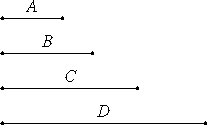# Proposition 7

If there are as many numbers as we please in continued proportion, and the first measures the last, then it also measures the second.

Let there are as many numbers as we please, A, B, C, and D, in continued proportion, and let A measure D.I say that A also measures B.

VIII.6

If A does not measure B, neither does any other of the numbers measure any other. But A measures D. Therefore A also measures B.

Therefore, if there are as many numbers as we please in continued proportion, and the first measures the last, then it also measures the second.

Q.E.D.

## Guide

This proposition is the contrapositive of the previous theorem.

#### Use of this theorem

This proposition is used in propositions VIII.14 and VIII.15.